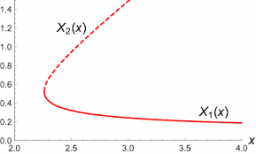# Substituted 65024

Which number should be substituted for the variable and in the equation:

4x2 (7-x) = a-3- (x-2), so that the root of the equation is the number 5?

a =  206

### Step-by-step explanation:Did you find an error or inaccuracy? Feel free to write us. Thank you!

Tips to related online calculators
Looking for help with calculating roots of a quadratic equation?
Do you have a linear equation or system of equations and looking for its solution? Or do you have a quadratic equation?